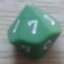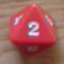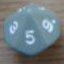Differently exploding dice

## Differently exploding dice

 Mon Feb 16 13:33:25 2015   by   Leichensack Hey folks,tar:= choose {2 .. 6};aq:= count tar = 7d6;res:= aq * tar;aq d6 + resThese lines actually explain the dice system better than words ever could, though there are unfinished business. They are supposed to simulate the following (ideally in a way where i could easily switch to another dice class):1. You pick and roll a single die, which defines your target (in case of 1 you roll again, until it's anything else).2. Then you take a look at your character sheet and take as many dice as you have skill points, let's just say you had 7 dice to roll.3. From those dice you only count those that are equal to your target. If you rolled three 4s with the target being 4 as well than you had 12 points for now.(3a. We'll let somebody who didn't gather any points by now reroll his dice once again with the target staying the same.)4. Then you were supposed to reroll those that had been fitting the target and add them up to your points. For our example this would mean you had 3 more dice to roll, which would turn out to be {1,4,4}, adding up with the former 12 making a result of 21.5. Another step (/Further steps?)  would be rolling the two 4s we got in step 4 again, sum up, and roll on until it's results are not equal to the target anymore. If we were to roll {2,4} in this step, we could be content with 27, yet there is one die left exploding and if that one came down as {3} we left this example with 30 Points.Distribution error: Singleton expected at line 4, column 7While i believe to be in the know of what the problem is, it seems to be too much for me at the moment to solve this problem all by myself.Any help in this matter would be greatly appreciated.best regards,the leichensack Mon Feb 16 15:27:44 2015   by   Leichensack Hey folks,tar:= choose {2 .. 6};aq:= count tar = 7d6;res:= aq * tar;aq d6 + resThese lines actually explain the dice system better than words ever could, though there are unfinished business. They are supposed to simulate the following (ideally in a way where i could easily switch to another dice class):1. You pick and roll a single die, which defines your target (in case of 1 you roll again, until it's anything else).2. Then you take a look at your character sheet and take as many dice as you have skill points, let's just say you had 7 dice to roll.3. From those dice you only count those that are equal to your target. If you rolled three 4s with the target being 4 as well than you had 12 points for now.(3a. We'll let somebody who didn't gather any points by now reroll his dice once again with the target staying the same.)4. Then you were supposed to reroll those that had been fitting the target and add them up to your points. For our example this would mean you had 3 more dice to roll, which would turn out to be {1,4,4}, adding up with the former 12 making a result of 21.5. Another step (/Further steps?)  would be rolling the two 4s we got in step 4 again, sum up, and roll on until it's results are not equal to the target anymore. If we were to roll {2,4} in this step, we could be content with 27, yet there is one die left exploding and if that one came down as {3} we left this example with 30 Points.Distribution error: Singleton expected at line 4, column 7While i believe to be in the know of what the problem is, it seems to be too much for me at the moment to solve this problem all by myself.Any help in this matter would be greatly appreciated.best regards,the leichensack Mon Feb 16 16:20:43 2015   by   Leichensack tar:= choose {2 .. 6};aq:= count tar = 7d6;\if aq = 0 then aq := count tar = 7d6;res:= aq * tar;\repeatrrld:= aq d6; res:= res + sum rrld;aq:= count tar = rrld;\while aq > 0resOkay, I made it this far, but am stuck now with the conditions and iterations, as the lines commented out might imply. The second try is pretty important because it lowers the chance of getting a result of 0 from 27.91 to a mere 7.79 (http://anydice.com/program/545c), but it does not seem to work that way. I tried some variants like leaving out the colon, not assingning to a variable at all, assigning the whole if-then-block to a variable.As for the iteration, use of the command accumulate seems to be a bright idea, but does not ideally fit my purpose here. Sorry for the double post mess up btw. Mon Feb 16 16:22:12 2015   by   Leichensack tar:= choose {2 .. 6};aq:= count tar = 7d6;\if aq = 0 then aq := count tar = 7d6;res:= aq * tar;\repeatrrld:= aq d6; res:= res + sum rrld;aq:= count tar = rrld;\while aq > 0resOkay, I made it this far, but am stuck now with the conditions and iterations, as the lines commented out might imply. The second try is pretty important because it lowers the chance of getting a result of 0 from 27.91 to a mere 7.79 (http://anydice.com/program/545c), but it does not seem to work that way. I tried some variants like leaving out the colon, not assingning to a variable at all, assigning the whole if-then-block to a variable.As for the iteration, use of the command accumulate seems to be a bright idea, but does not ideally fit my purpose here. Sorry for the double post mess up btw. Mon Feb 16 16:33:08 2015   by   Torben Starting with the error message, that is easy enough to explain:  When you write aq d6 you get a collection of aq values between 1 and 6, and since + is only defined on singleton collections, you get the error.  If you write sum aq d6 + res, the error should go away.The nonstandard explosion is more difficult.  The easiest way is to treat each die separately: Each die is rolled once.  If the result is not equal to the target, the result is 0.  If it is equal to the target, we add to this an exploding reroll of the die where the last result (which is not equal to the target) is also added.  We do this for all 7 dice and add the results.  We can express this as:`tar:= choose {2 .. 6};sum 7#(x := d6;      if x=tar then tar + sum accumulate v := d6 while v=tar      else 0)`Basically, each die is a straightforward exploding die, except that we count the first roll as 0 if it does not match the target.Your step 3a basically says that if the result of all dice is 0, you get to reroll.  It is not clear if you keep rerolling until you get a non-zero result or if it is only once.  If it is only once, we can handle this with a simple if-then-else:`tar:= choose {2 .. 6};result := sum 7#(x := d6;                if x=tar then tar + sum accumulate v := d6 while v=tar                else 0);if result = 0 then  sum 7#(x := d6;        if x=tar then tar + sum accumulate v := d6 while v=tar        else 0)else result`If we reroll until we get a non-zero result, we can do it with a repeat:`tar:= choose {2 .. 6};repeat  result := sum 7#(x := d6;                  if x=tar then tar + sum accumulate v := d6 while v=tar                  else 0)while result = 0`I hope this helps. Mon Feb 16 16:58:03 2015   by   Leichensack ... This time I shan't press f5 ...Yes, this seems to be it. I was stunned at how few lines it takes, and a little ashamed of that I only managed to contribute one line of code to the result. Yet if you switch out the approach completely it's kind of natural. Thank you very much, Sir.One question though: Is the Else in a if-then-else mandatory? Mon Feb 16 17:11:21 2015   by   Torben You are welcome.And, yes, the else is mandatory.